# numpy绘制方波及方波无穷级数的表示

2019/08/24 00:16

## 绘制方波

# 傅里叶级数是以正弦函数和余弦函数为基函数的无穷级数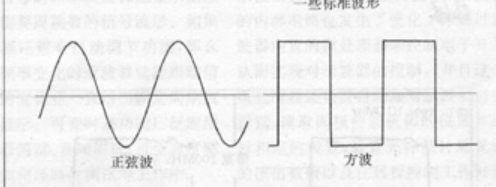### Code

import numpy as np
import matplotlib.pyplot as plt

t = np.linspace(-np.pi, np.pi, 201)
k = np.arange(1, 5)
k = 2 * k - 1
#k = 99
f = np.zeros_like(t)

for i in range(len(t)):
f[i] = np.sum(np.sin(k * t[i])/k)

f = (4 / np.pi) * f

plt.plot(t, f)
plt.show()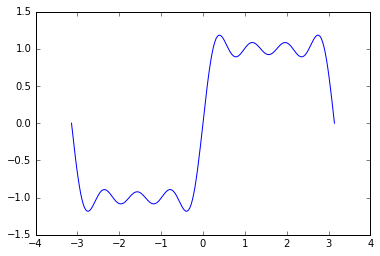# 方波的无穷级数表示

## 欧拉公式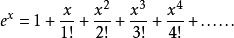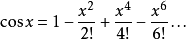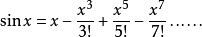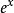的展开式中把x换成±ix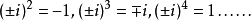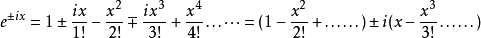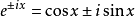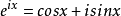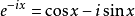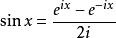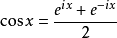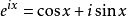中将x = π, 得出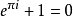## 傅里叶级数

# 频率是反映周期变化的快慢

# 只有e^x 和sinx、cosx的二阶导数是它们自身

1. 傅里叶展开就是把周期函数拆开成 常数（直流分量）+一倍频分量+2倍频分量+…

# 正弦函数和余弦函数的不同只是相位的不同

2.计算周期函数在各个倍频分量下的投影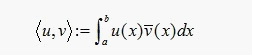3. 根据投影计算各倍频分量下的内积

4. 将这些内积求和

给定一个周期为T的函数x(t),那么它可以表示为无穷级数: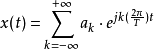j为虚数单位

2π/T 表示该分量的频率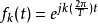表示x(t)的各个分量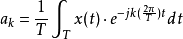表示函数x(t)在各个分量上的投影

ak * fk(t) 表示周期函数x(t)在某个分量下的内积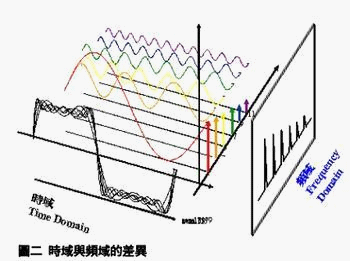1. 根据基频, 计算出各个分量

2. 计算周期函数在这些分量中的投影

3. 计算周期函数在各个分量下的内积, 并求和

0
0 收藏

### 作者的其它热门文章0 评论
0 收藏
0